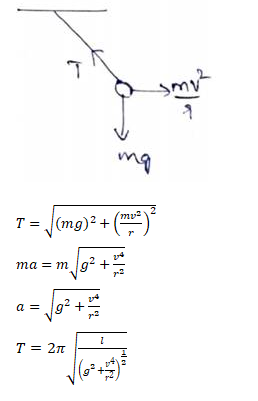# A simple pendulum of length I is suspended from

Question:

A simple pendulum of length $I$ is suspended from the ceiling of the car moving with a speed $v$ on a circular horizontal road of radius $\mathrm{r}$. (a) Find the tension in the string when it is at rest with respect to car. (b) Find the time period of small oscillation.

Solution: